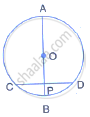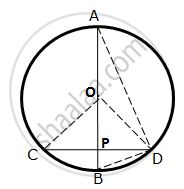Share

Books Shortlist

# A Chord Cd of a Circle Whose Centre is O, is Bisected at P by a Diameter Ab. Given Oa = Ob = 15 Cm and Op = 9 Cm. Calculate the Length Of: (I) Cd (Ii) Ad (Iii) Cb - ICSE Class 10 - Mathematics

ConceptChord Properties - the Perpendicular to a Chord from the Center Bisects the Chord (Without Proof)

#### Question

A chord CD of a circle whose centre is O, is bisected at P by a diameter AB.Given OA = OB = 15 cm and OP = 9 cm. calculate the length of:
(i) CD (ii) AD (iii) CB

#### SolutionOP⊥CD
∴ OP bisects CD
(Perpendicular drawn from the centre of a circle to a chord bisects it)

⇒ CP=("CD")/2

In right ΔOPC,

OC2 =OP2 + CP2

⇒ CP2= OC2 - OP2 =(15)2 - (9)2 = 144
∴ CP =12 cm
∴ CD =12×2 = 24 cm

(ii) Join BD
∴ BP = OB - OP = 15 - 9 = 6 cm
In right ΔBPD,
BD2 = BP2 + PD2

= (6)2 + ( 12)2 = 180

(Angle in a semicircle is a right angle)

∴ "AD" =sqrt 720 = 26.83 "cm"
(iii) Also, BC = BD sqrt 180 =13.42 cm